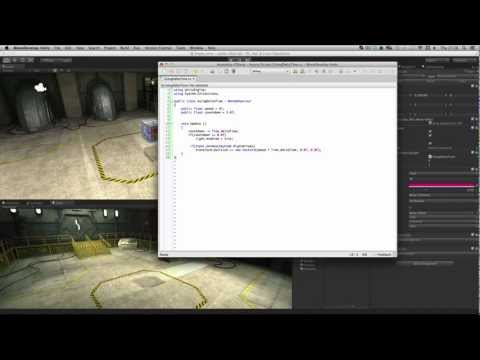# Delta Time

#### Difficulty: Beginner

What is Delta Time and how can it be used in your games to smooth and interpret values.## Delta Time

Beginner Scripting

### Code snippet

``````using UnityEngine;
using System.Collections;

public class UsingDeltaTime : MonoBehaviour
{
public float speed = 8f;
public float countdown = 3.0f;

void Update ()
{
countdown -= Time.deltaTime;
if(countdown <= 0.0f)
light.enabled = true;

if(Input.GetKey(KeyCode.RightArrow))
transform.position += new Vector3(speed * Time.deltaTime, 0.0f, 0.0f);
}
}``````
``````#pragma strict

public var speed : float = 8f;
public var countdown : float = 3f;

function Update ()
{
countdown -= Time.deltaTime;
if(countdown <= 0.0f)
renderer.material.color = Color.red;

if(Input.GetKey(KeyCode.RightArrow))
transform.position += new Vector3(speed * Time.deltaTime, 0.0f, 0.0f);

}``````
``````import UnityEngine
import System.Collections

public class UsingDeltaTimes(MonoBehaviour):

public speed as single = 8.0F
public countdown as single = 3.0F

private def Update():
countdown -= Time.deltaTime
if countdown <= 0.0F:
renderer.material.color = Color.red
if Input.GetKey(KeyCode.RightArrow):
transform.position += Vector3((speed * Time.deltaTime), 0.0F, 0.0F)``````

#### Related documentation